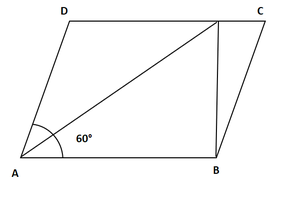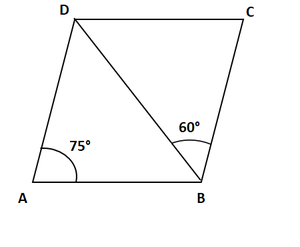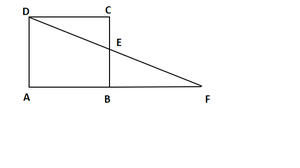# Class 9 RD Sharma Solutions – Chapter 14 Quadrilaterals – Exercise 14.2

• Last Updated : 28 Mar, 2021

### Question 1. Two opposite angles of a parallelogram are (3x – 2)° and (50 – x)°. Find the measure of each angle of the parallelogram.

Solution:

Given: Two opposite angles of a parallelogram are (3x – 2)° and (50 – x)°.

Attention reader! All those who say programming isn't for kids, just haven't met the right mentors yet. Join the  Demo Class for First Step to Coding Coursespecifically designed for students of class 8 to 12.

The students will get to learn more about the world of programming in these free classes which will definitely help them in making a wise career choice in the future.

(3x – 2)°= (50 – x)° [Opposite sides of a parallelogram are equal]3x + x = 50 + 24x = 52x = 13

Angle x is 13°(3x – 2) = (3*13 – 2) = 37°

(50 – x)° = (50 – 13)°= 37°x + 37°= 180° [Adjacent angles of a parallelogram are supplementary]x = 180°− 37°= 143°

Hence, required angles are : 37°, 143°, 37°, 143°.

### Question 2. If an angle of a parallelogram is two-third of its adjacent angle, find the angles of the parallelogram.

Solution:

Let the measure of the angle be x.

Therefore, the measure of the angle adjacent is 2x/3

Hence, x + 2x/3 = 180° [Consecutive angles of a parallelogram are supplementary]

2x + 3x = 540°5x = 540°x = 108°

Now,

⟹ x + 108°= 180° [Consecutive angles of a parallelogram are supplementary]

⟹ x + 108°= 180°

⟹ x = 180°- 108°= 72°

⟹ x = 72°required angles are180°, 72°, 180°, 72°

### Question 3. Find the measure of all the angles of a parallelogram, if one angle is 24°less than twice the smallest angle.

Solution:

x + 2x – 24°= 180° [Consecutive angles of a parallelogram are supplementary]3x – 24°= 180°3x = 108° + 24°3x = 204°x = 204/3 = 68°x = 68°

Other angle of parallelogram=2x – 24°= 2*68°- 24°= 112°required angles are 68°,112°,68°,112°

### Question 4. The perimeter of a parallelogram is 22 cm. If the longer side measures 6.5 cm what is the measure of the shorter side?

Solution:

Given: perimeter of a parallelogram is 22 cm

Let us consider the shorter side be ‘y’.perimeter = y + 6.5 + 6.5 + x [Sum of all sides]

22 = 2(y + 6.5)

11 = y + 6.5y = 11 – 6.5 = 4.5 cm

Hence, the measure of the shorter side = 4.5 cm

### Question 5. In a parallelogram ABCD, ∠D = 135°. Determine the measures of ∠A and ∠B.

Solution:

In a parallelogram ABCD

Given: ∠D=135°

So, ∠D + ∠C = 180° [Consecutive angles of a parallelogram are supplementary]

∠C = 180°− 135°

∠C = 45°

In a parallelogram opposite sides are equal.

∠A = ∠C = 45° [opposite sides of parallelogram are equal]

∠B = ∠D = 135°

hence, the measures of ∠A and ∠B are 45°,135°respectively.

### Question 6. ABCD is a parallelogram in which ∠A = 70°. Compute ∠B, ∠C and ∠D.

Solution:

In a parallelogram ABCD

Given: ∠A = 70°

∠A + ∠B = 180° [Consecutive angles of a parallelogram are supplementary]

70°+ ∠B = 180° [given ∠A = 70°]

∠B = 180°− 70°

∠B = 110°

Now,

∠A = ∠C = 70° [opposite sides of parallelogram are equal]

∠B = ∠D = 110°

hence, the measures of ∠A and ∠B are 70°,110°respectively.

### Question 7. In Figure, ABCD is a parallelogram in which ∠A = 60°. If the bisectors of ∠A, and ∠B meet at P, prove that AD = DP, PC = BC and DC = 2AD.

Solution:Given: ∠A = 60°

To prove: AD = DP, PC = BC and DC = 2AD

AP bisects ∠A

so, ∠DAP = ∠PAB = 30°

Now,

∠A + ∠B = 180° [Consecutive angles of a parallelogram are supplementary]

∠B + 60°= 180°

∠B = 180°− 60°

∠B = 120°

BP bisects ∠B

so, ∠PBA = ∠PBC = 60°

∠PAB = ∠APD = 30°[Alternate interior angles]

Therefore, AD = DP [Sides opposite to equal angles are in equal length]

Similarly

∠PBA = ∠BPC = 60° [Alternate interior angles]

Therefore, PC = BC

DC = DP + PC

DC = AD + BC [ DP = AD and PC = BC ]

DC = 2AD [Since, AD = BC, The opposite sides of parallelogram are parallel and congruent]

hence proved.

### Question 8. In figure, ABCD is a parallelogram in which ∠DAB = 75°and ∠DBC = 60°. Compute ∠CDB, and ∠ADB.

Solution:Given: ∠DAB = 75°and ∠DBC = 60°

∠CBD = ∠ADB = 60° [Alternate interior angle. AD∥ BC and BD is the transversal]

InBDA

∠DAB + ∠ADB + ∠ABD = 180° [Angle sum property]75°+ 60°+ ∠CDB = 180°∠ABD = 180°− (135°)∠ABD = 45°

∠ABD = ∠CDB = 45° [Alternate interior angle. AD∥ BC and BD is the transversal]

Hence, ∠CDB = 45°, ∠ADB = 60°

### Question 9. In figure, ABCD is a parallelogram and E is the mid-point of side BC. If DE and AB when produced meet at F, prove that AF = 2AB.

Solution:Given: ABCD is a parallelogram and E is the mid-point of side BC.

To prove: AF = 2AB.

Now,

In ΔBEF and ΔCED

∠BEF = ∠CED [Verified opposite angle]

BE = CE [Since, E is the mid-point of BC]

∠EBF = ∠ECD [Since, Alternate interior angles are equal]ΔBEF ≅ ΔCED [ASA congruence]BF = CD [Corresponding Parts of Congruent Triangle]

AF = AB + AF

AF = AB + CD [BF = CD by Corresponding Parts of Congruent Triangle ]

AF = AB + AB [CD=AB, The opposite sides of parallelogram are parallel and congruent]

AF = 2AB.

Hence proved.

### Question 10. Which of the following statements are true (T) and which are false (F)?

(i) In a parallelogram, the diagonals are equal.

(ii) In a parallelogram, the diagonals bisect each other.

(iii) In a parallelogram, the diagonals intersect each other at right angles.

(iv) In any quadrilateral, if a pair of opposite sides is equal, it is a parallelogram.

(v) If all the angles of a quadrilateral are equal, it is a parallelogram.

(vi) If three sides of a quadrilateral are equal, it is a parallelogram.

(vii) If three angles of a quadrilateral are equal, it is a parallelogram.

(viii) If all the sides of a quadrilateral are equal, it is a parallelogram.

Solution:

(i) False

(ii) True

(iii) False

(iv) False

(v) True

(vi) False

(vii) False

(viii) True

My Personal Notes arrow_drop_up